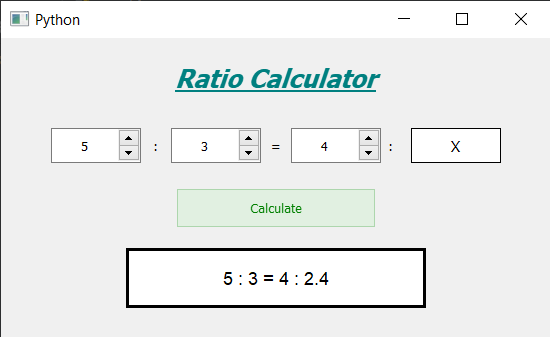# Ratio Calculator using PyQt5

• Last Updated : 10 Jul, 2020

In this article we will see how we can create a ratio calculator using PyQt5. Ratio is a contrast, which exists between two particular numbers, is defined as ratio. This ratio calculator is developed to compute this contrast and find out the relationship between these numbers. Below is how the calculator will lookAttention geek! Strengthen your foundations with the Python Programming Foundation Course and learn the basics.

To begin with, your interview preparations Enhance your Data Structures concepts with the Python DS Course. And to begin with your Machine Learning Journey, join the Machine Learning - Basic Level Course

PyQt5 is cross-platform GUI toolkit, a set of python bindings for Qt v5. One can develop an interactive desktop application with so much ease because of the tools and simplicity provided by this library. Below is the command to install the PyQt5

`pip install PyQt5`

Concept :
User has to select a ratio then enter another number for which calculator will find the corresponding ratio value, below is the formula used

```A / B = C / X
```

Here A and B are the selected ratio and C is the entered number for which we have to find corresponding value of the ratio

GUI Implementation Steps :
1. Create a heading label that display the calculator name
2. Create three spin box for getting ‘A’, ‘B’ and ‘C’ values
3. Create labels between spin boxes for ratio and equal to symbol and label for showing ‘X’ value
4. Create push button for calculate the ratio value
5. Create a label to show the calculated value

Back-End Implementation :
1. Set range to each of the spin box with minimum value equal to 1 so that user can’t enter 0 as input
2. Set various properties like alignment, geometry to each of the widget in the window
3. Add color effect to the push button
4. Add action tot he push button when it get clicked
5. Inside the push button action calculate the value of ‘X’ according to the values entered by user
6. Remove decimal values if it is a whole number
7. Show the output with the help of result label

Below is the implementation

 `# importing libraries``from` `PyQt5.QtWidgets ``import` `*` `from` `PyQt5 ``import` `QtCore, QtGui``from` `PyQt5.QtGui ``import` `*` `from` `PyQt5.QtCore ``import` `*` `import` `sys`` ` ` ` `class` `Window(QMainWindow):`` ` `    ``def` `__init__(``self``):``        ``super``().__init__()`` ` `        ``# setting title``        ``self``.setWindowTitle(``"Python "``)`` ` `        ``# width of window``        ``self``.w_width ``=` `550`` ` `        ``# height of window``        ``self``.w_height ``=` `300`` ` `        ``# setting geometry``        ``self``.setGeometry(``100``, ``100``, ``self``.w_width, ``self``.w_height)`` ` `        ``# calling method``        ``self``.UiComponents()`` ` `        ``# showing all the widgets``        ``self``.show()`` ` `    ``# method for components``    ``def` `UiComponents(``self``):`` ` `        ``# creating head label``        ``head ``=` `QLabel(``"Ratio Calculator"``, ``self``)`` ` `        ``head.setWordWrap(``True``)`` ` `        ``# setting geometry to the head``        ``head.setGeometry(``0``, ``10``, ``550``, ``60``)`` ` `        ``# font``        ``font ``=` `QFont(``'Times'``, ``15``)``        ``font.setBold(``True``)``        ``font.setItalic(``True``)``        ``font.setUnderline(``True``)`` ` `        ``# setting font to the head``        ``head.setFont(font)`` ` `        ``# setting alignment of the head``        ``head.setAlignment(Qt.AlignCenter)`` ` `        ``# setting color effect to the head``        ``color ``=` `QGraphicsColorizeEffect(``self``)``        ``color.setColor(Qt.darkCyan)``        ``head.setGraphicsEffect(color)`` ` `        ``# creating a spin box``        ``self``.a_spin ``=` `QSpinBox(``self``)`` ` `        ``# setting geometry``        ``self``.a_spin.setGeometry(``50``, ``90``, ``90``, ``35``)`` ` `        ``# setting alignment``        ``self``.a_spin.setAlignment(Qt.AlignCenter)`` ` `        ``# setting range``        ``self``.a_spin.setRange(``1``, ``999999``)`` ` `        ``# creating a label``        ``l1 ``=` `QLabel(``" : "``, ``self``)`` ` `        ``# setting geometry``        ``l1.setGeometry(``140``, ``90``, ``30``, ``35``)`` ` `        ``# setting alignment``        ``l1.setAlignment(Qt.AlignCenter)`` ` `        ``# creating a spin box``        ``self``.b_spin ``=` `QSpinBox(``self``)`` ` `        ``# setting geometry``        ``self``.b_spin.setGeometry(``170``, ``90``, ``90``, ``35``)`` ` `        ``# setting alignment``        ``self``.b_spin.setAlignment(Qt.AlignCenter)`` ` `        ``# setting range``        ``self``.b_spin.setRange(``1``, ``999999``)`` ` `        ``# creating a label``        ``l2 ``=` `QLabel(``" = "``, ``self``)`` ` `        ``# setting geometry``        ``l2.setGeometry(``260``, ``90``, ``30``, ``35``)`` ` `        ``# setting alignment``        ``l2.setAlignment(Qt.AlignCenter)`` ` `        ``# creating a spin box``        ``self``.c_spin ``=` `QSpinBox(``self``)`` ` `        ``# setting geometry``        ``self``.c_spin.setGeometry(``290``, ``90``, ``90``, ``35``)`` ` `        ``# setting alignment``        ``self``.c_spin.setAlignment(Qt.AlignCenter)`` ` `        ``# setting range``        ``self``.c_spin.setRange(``1``, ``999999``)`` ` `        ``# creating a label``        ``l3 ``=` `QLabel(``" : "``, ``self``)`` ` `        ``# setting geometry``        ``l3.setGeometry(``380``, ``90``, ``20``, ``35``)`` ` `        ``# setting alignment``        ``l3.setAlignment(Qt.AlignCenter)`` ` `        ``# creating a label``        ``lx ``=` `QLabel(``"X"``, ``self``)`` ` `        ``# setting geometry``        ``lx.setGeometry(``410``, ``90``, ``90``, ``35``)`` ` `        ``# setting alignment``        ``lx.setAlignment(Qt.AlignCenter)`` ` `        ``# setting style sheet``        ``lx.setStyleSheet(``"QLabel"``                         ``"{"``                         ``"border : 1px solid black;"``                         ``"background-color : white;"``                         ``"font-size : 15px;"``                         ``"}"``)`` ` `        ``# creating a push button``        ``calculate ``=` `QPushButton(``"Calculate"``, ``self``)`` ` `        ``# setting geometry to the push button``        ``calculate.setGeometry(``175``, ``150``, ``200``, ``40``)`` ` `        ``# adding action to the button``        ``calculate.clicked.connect(``self``.calculate)`` ` `        ``# adding color effect to the push button``        ``color ``=` `QGraphicsColorizeEffect()``        ``color.setColor(Qt.darkGreen)``        ``calculate.setGraphicsEffect(color)`` ` `        ``# creating a label to show result``        ``self``.result ``=` `QLabel(``self``)`` ` `        ``# setting properties to result label``        ``self``.result.setAlignment(Qt.AlignCenter)`` ` `        ``# setting geometry``        ``self``.result.setGeometry(``125``, ``210``, ``300``, ``60``)`` ` `        ``# making it multi line``        ``self``.result.setWordWrap(``True``)`` ` `        ``# setting stylesheet``        ``# adding border and background``        ``self``.result.setStyleSheet(``"QLabel"``                                  ``"{"``                                  ``"border : 3px solid black;"``                                  ``"background : white;"``                                  ``"}"``)`` ` `        ``# setting font``        ``self``.result.setFont(QFont(``'Arial'``, ``11``))`` ` ` ` `    ``def` `calculate(``self``):`` ` `        ``# getting spin box 'a' value``        ``a ``=` `self``.a_spin.value()`` ` `        ``# getting spin box 'b' value``        ``b ``=` `self``.b_spin.value()`` ` `        ``# getting spin box 'c value``        ``c ``=` `self``.c_spin.value()`` ` `        ``# calculating 'x' value``        ``x ``=` `(c ``*` `b) ``/` `a`` ` `        ``# removing decimal if decimal is zero``        ``if` `x ``%` `1` `=``=` `0``:``            ``x ``=` `int``(x)`` ` ` ` `        ``# setting text to the result label``        ``self``.result.setText(``str``(a) ``+` `" : "` `+` `str``(b) ``+` `" = "``                            ``+` `str``(c) ``+` `" : "` `+` `str``(x))`` ` ` ` ` ` ` ` ` ` `# create pyqt5 app``App ``=` `QApplication(sys.argv)`` ` `# create the instance of our Window``window ``=` `Window()`` ` `# start the app``sys.exit(App.``exec``())`

Output :

My Personal Notes arrow_drop_up